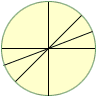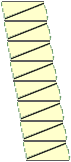Level of problems: 1st year college.

I have two problems.

The first: prove that the area of a cirlce is pi times radius squared without using calculus.

The second: show that ln(x) < x - 1 for all x > 0.

 One "proof" that the area of a cirlce is pi times radius squared starts by cutting the top half of the circle into sectors of equal size. In the diagram I have started to cut it into sectors with an angle of pi by 8 radians (22.5o). Next cut up the bottom half exactly the same way. Now take the sectors and stack them as in the second diagram. If the number of sectors is large this stack is approximately a rectangle with base the radius of the circle and height one half the circumference of the circle.    One way to approach the second problem is to let f(x) = (1 + ln(x))/x and use calculus to show that for x > 0 the minimum value of f(x) is 1. Cheers Harley